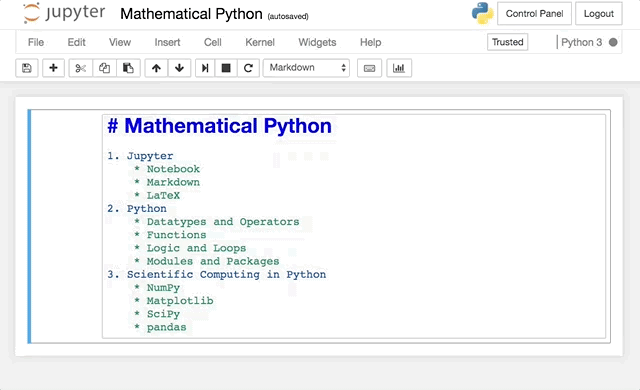# Markdown

Markdown is a simple text-to-HTML markup language written in plain text. Jupyter notebook recognizes markdown and renders markdown code as HTML. See Markdown (by John Gruber) and GitHub Markdown Help for more information.## Text

Output Syntax
emphasis *emphasis*
strong **strong**
code code

Output Syntax

# Heading 1

## Heading 2

### Heading 3

#### Heading 4
##### Heading 5
###### Heading 6

## Lists

Create an ordered list using numbers:

1. Number theory
2. Algebra
3. Partial differential equations
4. Probability

1. Number theory
2. Algebra
3. Partial differential equations
4. Probability

Create an unordered list using an asterisk * for each item:

* Number theory
* Algebra
* Partial differential equations
* Probability

• Number theory
• Algebra
• Partial differential equations
• Probability

Use indentation to create nested lists:

1. Mathematics
* Calculus
* Linear Algebra
* Probability
2. Physics
* Classical Mechanics
* Relativity
* Thermodynamics
3. Biology
* Diffusion and Osmosis
* Homeostasis
* Immunology

1. Mathematics
• Calculus
• Linear Algebra
• Probability
2. Physics
• Classical Mechanics
• Relativity
• Thermodynamics
3. Biology
• Diffusion and Osmosis
• Homeostasis
• Immunology

Create a link with the syntax [description](url). For example:

[UBC Math](http://www.math.ubc.ca)


## Images

Include an image using the syntax ![description](url). For example:

![Jupyter logo](https://jupyter.org/assets/try/jupyter.png)


displays the image## Tables

Create a table by separating entries by pipe characters |:

| Python Operator | Description  |
| :---: | :---: |
| + | addition |
| - | subtraction |
| * | multiplication |
| / | division |
| ** | power |

Python Operator Description
+ addition
- subtraction
* multiplication
/ division
** power

The syntax :---: specifies the alignment (centered in this case) of the columns. See more about GitHub flavoured markdown.

## Exercises

Exercise 1. Create a numbered list of the top 5 websites you visit most often and include a link for each site.

Exercise 2. Write a short biography of your favourite mathematician, provide a link to their Wikipedia page and include an image (with a link and description of the source).

Exercise 3. Create a table of all the courses that you have taken in university. Include the columns: course number, course title, year (that you took the class), and instructor name.